# NCERT Solutions for Class 11 Physics chapter-5 Laws of Motion

## NCERT Solutions for Class 11 Physics

NCERT Solutions for class-11 Physics Chapter-5 Laws of Motion is prepared by our senior and renowned teachers of pw primary focus while solving these questions of class-11 in NCERT textbook, also do read theory of this Chapter-5 Laws of Motion while going before solving the NCERT questions. You can download NCERT solution of all chapters from Physics Wallah in PDF.

### Chapter-5 Laws of Motion

Question1. Give the magnitude and direction of the net force acting on
a. a drop of rain falling down with a constant speed,
b. a cork of mass 10 g floating on water,
c. a kite skillfully held stationary in the sky,
d. a car moving with a constant velocity of 30 km/h on a rough road,
e. a high-speed electron in space far from all material objects, and free of electric and magnetic fields.

Solution :
a. As the rain drop is flling with a constant speed, its accleration, a = 0. Hence net force F= ma  = 0.
b. As the cork is floating on water, its weight is balanced by the upthrust due to water. Therefore, the net force on the cork is 0.
c. As the kite is held stationery, in accordance with the first law of motion, the net force on the kite is 0.
d. Force is being applied to overcome the force of friction. But as velocity of the car is constant, its accleleration, a = 0. Hence net force on the car F = ma = 0.
e. As the high speed electron in space is far away from all gravitating objects and free of electric and magnetic fields, the net force on electron is 0.

Question2. A pebble of mass 0.05 kg is thrown vertically upwards. Give the direction and magnitude of the net force on the pebble,
a. during its upward motion,
b. during its downward motion,
c. at the highest point where it is momentarily at rest. Do your answers change if the pebble was thrown at an angle of 45° with the horizontal direction?
Ignore air resistance.

Solution :
0.5 N, in vertically downward direction, in all cases
Acceleration due to gravity, irrespective of the direction of motion of an object, always acts downward. The gravitational force is the only force that acts on the pebble in all three cases. Its magnitude is given by Newton’s second law of motion as:
F = m × a
Where,
F = Net force
m = Mass of the pebble = 0.05 kg
a = g = 10 m/s2
∴F = 0.05 × 10 = 0.5 N
The net force on the pebble in all three cases is 0.5 N and this force acts in the downward direction.
If the pebble is thrown at an angle of 45° with the horizontal direction, it will have both the horizontal and vertical components of velocity. At the highest point, only the vertical component of velocity becomes zero. However, the pebble will have the horizontal component of velocity throughout its motion. This component of velocity produces no effect on the net force acting on the pebble.

Question3. Give the magnitude and direction of the net force acting on a stone of mass 0.1 kg,
a.  just after it is dropped from the window of a stationary train,
b. just after it is dropped from the window of a train running at a constant velocity of 36 km/h,
c. just after it is dropped from the window of a train accelerating with 1 m s-2,
d. lying on the floor of a train which is accelerating with 1 m s-2, the stone being at rest relative to the train. Neglect air resistance throughout.

Solution :
a. Here, m = 0.1 Kg, a = + g = 10 m/s2
Net force, F = ma = 0.1 × 10 = 1.0 N
This forcer acts vertically downwards.

b. When the train is running at a constant velocity, its acceleration = 0, No force acts on the stone due to this motion. Therefore, force on the stone F = weight of stone = mg = 0.1 × 10 = 1.0 N
This force also acts vertically downwards.

c. When the train is accelerating with 1 m s-2, an additional force F' = ma = 0.1 × 1 = 0.1 N acts on the stone in the horizontal direction. But once the stone is dropped from the train, F' becomes zero and the net force on the stone is F = mg = 0.1 × 10 = 1.0 N, acting vertically downwards.

d. As the stone is lying on the floor of the trin, its acceleration is same as that of the train.
∴ force acting on stone, F = ma = 0.1 × 1 = 0.1 NThis force is along the horizontal direction of motion of the train.
Note that in each case, the weight of the stone is being balanced by the normal reaction.

Question4. One end of a string of length l is connected to a particle of mass m and the other to a small peg on a smooth horizontal table. If the particle moves in a circle with speed v the net force on the particle (directed towards the centre) is:
(i) T, (ii) T - mv2 / l, (iii) T + mv2 / l, (iv) 0
T is the tension in the string. [Choose the correct alternative].

Solution :
(i) T
When a particle connected to a string revolves in a circular path around a centre, the centripetal force is provided by the tension produced in the string. Hence, in the given case, the net force on the particle is the tension T, i.e.,
F = T = mv2 / l
Where F is the net force acting on the particle.

Question5. A constant retarding force of 50 N is applied to a body of mass 20 kg moving initially with a speed of 15 ms–1. How long does the body take to stop?

Solution :
Retarding force, F = –50 N
Mass of the body, m = 20 kg
Initial velocity of the body, u = 15 m/s
Final velocity of the body, v = 0
Using Newton’s second law of motion, the acceleration (a) produced in the body can be calculated as:
F = ma
–50 = 20 × a
∴ a = -50/20 = -2.5 ms-2
Using the first equation of motion, the time (t) taken by the body to come to rest can be calculated as:
v = u + at
∴ t = -u / a = -15 / -2.5 = 6 s

Question 6. A constant force acting on a body of mass 3.0 kg changes its speed from 2.0 m s–1 to 3.5 m s–1 in 25 s. The direction of the motion of the body remains unchanged. What is the magnitude and direction of the force?

Solution :
Mass of the body, m = 3 kg
Initial speed of the body, u = 2 m/s
Final speed of the body, v = 3.5 m/s
Time, t = 25 s
Using the first equation of motion, the acceleration (a) produced in the body can be calculated as:
v = u + at
∴ a = (v - u) / t
= (3.5 - 2) / 25 = 0.06 ms-2
As per Newton’s second law of motion, force is given as:
F = ma
= 3 × 0.06 = 0.18 N
Since the application of force does not change the direction of the body, the net force acting on the body is in the direction of its motion.

Question7. A body of mass 5 kg is acted upon by two perpendicular forces 8 N and 6 N. Give the magnitude and direction of the acceleration of the body.

Solution :
Mass of the body, m = 5 kg
The given situation can be represented as follows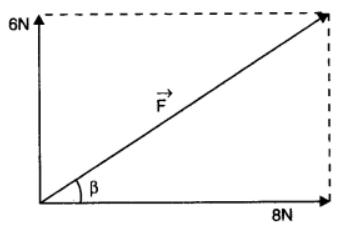The resultant of two forces is given as: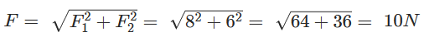θ is the angle made by R with the force of 8 N
∴ θ = tan-1(-6/8) = -36.870
The negative sign indicates that θ is in the clockwise direction with respect to the force of magnitude 8 N.

As per Newton’s second law of motion, the acceleration (a) of the body is given as:
F = ma
∴ a = F / m = 10 / 5 = 2 ms-2

Question 8. The driver of a three-wheeler moving with a speed of 36 km/h sees a child standing in the middle of the road and brings his vehicle to rest in 4.0 s just in time to save the child. What is the average retarding force on the vehicle? The mass of the three-wheeler is 400 kg and the mass of the driver is 65 kg.

Solution :
Initial speed of the three-wheeler, u = 36 km/h = 10 m/s
Final speed of the three-wheeler, v = 0 m/s
Time, t = 4 s
Mass of the three-wheeler, m = 400 kg
Mass of the driver, m' = 65 kg
Total mass of the system, M = 400 + 65 = 465 kg
Using the first law of motion, the acceleration (a) of the three-wheeler can be calculated as:
v = u + at
∴ a = (v - u) / t = (0 - 10) / 4 = -2.5 ms-2
The negative sign indicates that the velocity of the three-wheeler is decreasing with time.
Using Newton’s second law of motion, the net force acting on the three-wheeler can be calculated as:
F = Ma
= 465 × (–2.5) = –1162.5 N
The negative sign indicates that the force is acting against the direction of motion of the three-wheeler.

Question 9. A rocket with a lift-off mass 20,000 kg is blasted upwards with an initial acceleration of 5.0 m s–2. Calculate the initial thrust (force) of the blast.

Solution :
Mass of the rocket, m = 20,000 kg
Initial acceleration, a = 5 m/s2
Acceleration due to gravity, g = 10 m/s2
Using Newton’s second law of motion, the net force (thrust) acting on the rocket is given by the relation:
F – mg = ma
F = m (g + a)
= 20000 × (10 + 5)
= 20000 × 15 = 3 × 105 N

Question 10. A body of mass 0.40 kg moving initially with a constant speed of 10 m s–1 to the north is subject to a constant force of 8.0 N directed towards the south for 30 s. Take the instant the force is applied to be t = 0, the position of the body at that time to be x = 0, and predict its position at t = –5 s, 25 s, 100 s

Solution :
Mass of the body, m = 0.40 kg
Initial speed of the body, u = 10 m/s due north
Force acting on the body, F = –8.0 N
Acceleration produced in the body, a = F / m = -8.0 / 0.40 = -20 ms-2

(i) At t = –5 s
Acceleration, a' = 0 and u = 10 m/s
s = ut + (1/2) a' t2
= 10 × (–5) = –50 m

(ii) At t = 25 s
Acceleration, a'' = –20 m/s2 and u = 10 m/s
s' = ut' + (1/2) a" t2
= 10 × 25 + (1/2) × (-20) × (25)2
= 250 - 6250 = -6000 m

(iii) At t = 100 s
For 0 ≤ t ≤ 30 s
a = -20 ms-2
u = 10 m/s
s1 = ut + (1/2)a"t2
= 10 × 30 + (1/2) × (-20) × (30)2
= 300 - 9000  =  -8700 m
For 30 < t ≤ 100 s

As per the first equation of motion, for t = 30 s, final velocity is given as:
v = u + at
= 10 + (–20) × 30 = –590 m/s
Velocity of the body after 30 s = –590 m/s
For motion between 30 s to 100 s, i.e., in 70 s:
s2 = vt + (1/2) a" t2
= -590 × 70 = -41300 m
∴ Total distance, s" = s1 + s2 = -8700 -41300 = -50000 m = -50 km.

Question 11. A truck starts from rest and accelerates uniformly at 2.0 m s–2. At t = 10 s, a stone is dropped by a person standing on the top of the truck (6 m high from the ground). What are the (a) velocity, and (b) acceleration of the stone at t = 11 s? (Neglect air resistance.)

Solution :
(a) Initial velocity of the truck, u = 0
Acceleration, a = 2 m/s2
Time, t = 10 s
As per the first equation of motion, final velocity is given as:
v = u + at
= 0 + 2 × 10 = 20 m/s
The final velocity of the truck and hence, of the stone is 20 m/s.
At t = 11 s, the horizontal component (vx) of velocity, in the absence of air resistance, remains unchanged, i.e.,
vx = 20 m/s
The vertical component (vy) of velocity of the stone is given by the first equation of motion as:
vy = u + ayδt
Where, δt = 11 – 10 = 1 s and ay = g = 10 m/s2
∴vy = 0 + 10 × 1 = 10 m/s
The resultant velocity (v) of the stone is given as: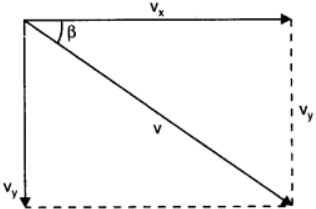v = (vx2 + vy2)1/2
= (202 + 102)1/2
= 22.36 m/s

Let θ be the angle made by the resultant velocity with the horizontal component of velocity, vx
∴ tan θ = (vy / vx)
θ = tan-1 (10 / 20)
= 26.570

(b) When the stone is dropped from the truck, the horizontal force acting on it becomes zero. However, the stone continues to move under the influence of gravity. Hence, the acceleration of the stone is 10 m/s2 and it acts vertically downward.

Question 12. A bob of mass 0.1 kg hung from the ceiling of a room by a string 2 m long is set into oscillation. The speed of the bob at its mean position is 1 m s–1. What is the trajectory of the bob if the string is cut when the bob is (a) at one of its extreme positions, (b) at its mean position.

Solution :
(a) Vertically downward
At the extreme position, the velocity of the bob becomes zero. If the string is cut at this moment, then the bob will fall vertically on the ground.

(b) Parabolic path
At the mean position, the velocity of the bob is 1 m/s. The direction of this velocity is tangential to the arc formed by the oscillating bob. If the bob is cut at the mean position, then it will trace a projectile path having the horizontal component of velocity only. Hence, it will follow a parabolic path.

Question 13. A man of mass 70 kg stands on a weighing scale in a lift which is moving
(a) upwards with a uniform speed of 10 m s–1,
(b) downwards with a uniform acceleration of 5 m s–2,
(c) upwards with a uniform acceleration of 5 m s–2.
What would be the readings on the scale in each case?
(d) What would be the reading if the lift mechanism failed and it hurtled down freely under gravity?

Solution :
(a) Mass of the man, m = 70 kg
Acceleration, a = 0
Using Newton’s second law of motion, we can write the equation of motion as:
R – mg = ma
Where, ma is the net force acting on the man.
As the lift is moving at a uniform speed, acceleration a = 0
∴ R = mg
= 70 × 10 = 700 N
∴ Reading on the weighing scale = 700 / g = 700 / 10 = 70 kg

(b) Mass of the man, m = 70 kg
Acceleration, a = 5 m/s2 downward
Using Newton’s second law of motion, we can write the equation of motion as:
R + mg = ma
R = m(g – a)
= 70 (10 – 5) = 70 × 5
= 350 N
∴ Reading on the weighing scale = 350 g = 350 / 10 = 35 kg

(c) Mass of the man, m = 70 kg
Acceleration, a = 5 m/s2 upward
Using Newton’s second law of motion, we can write the equation of motion as:
R – mg = ma
R = m(g + a)
= 70 (10 + 5) = 70 × 15
= 1050 N
∴ Reading on the weighing scale = 1050 / g = 1050 / 10 = 105 kg

(d) When the lift moves freely under gravity, acceleration a = g
Using Newton’s second law of motion, we can write the equation of motion as:
R + mg = ma
R = m(g – a)
= m(g – g) = 0
∴ Reading on the weighing scale = 0 / g = 0 kg
The man will be in a state of weightlessness.

Question 14. Figure 5.16 shows the position-time graph of a particle of mass 4 kg. What is the (a) force on the particle for t < 0, t > 4 s,0 < t < 4 s? (b) impulse at t = 0 and t = 4 s? (Consider one-dimensional motion only).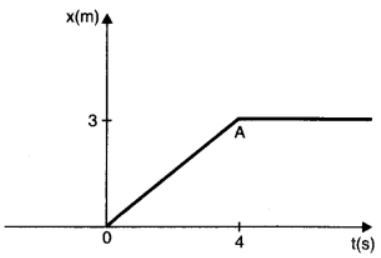Solution :
(a) For t < 0
It can be observed from the given graph that the position of the particle is coincident with the time axis. It indicates that the displacement of the particle in this time interval is zero. Hence, the force acting on the particle is zero.

For t > 4 s
It can be observed from the given graph that the position of the particle is parallel to the time axis. It indicates that the particle is at rest at a distance of
3 m from the origin. Hence, no force is acting on the particle.

For 0 < t < 4
It can be observed that the given position-time graph has a constant slope. Hence, the acceleration produced in the particle is zero. Therefore, the force acting on the particle is zero.

(b) At t = 0
Impulse = Change in momentum
= mv – mu
Mass of the particle, m = 4 kg
Initial velocity of the particle, u = 0
Final velocity of the particle, v = 3 / 4 m/s
∴ Impulse = 4 ( 3/4 - 0) = 3 kg m/s

At t = 4 s
Initial velocity of the particle, u = 3 / 4 m/s
Final velocity o9f the particle, v = 0
∴ Impulse = 4 (0 - 3/4) = -3 kg m/s

Question 15. Two bodies of masses 10 kg and 20 kg respectively kept on a smooth, horizontal surface are tied to the ends of a light string. A horizontal force F = 600 N is applied to (i) A, (ii) B along the direction of string. What is the tension in the string in each case?

Solution :
Horizontal force, F = 600 N
Mass of body A, m1 = 10 kg
Mass of body B, m2 = 20 kg
Total mass of the system, m = m1 + m2 = 30 kg
Using Newton’s second law of motion, the acceleration (a) produced in the system can be calculated as:
F = ma
∴ a = F / m  =  600 / 30  =  20 ms-2
When force F is applied on body A: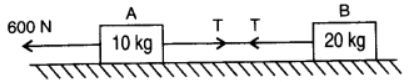The equation of motion can be written as:
F – T = m1a
∴T = F – m1a
= 600 – 10 × 20 = 400 N … (i)

When force F is applied on body B: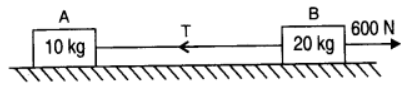The equation of motion can be written as:
F – T = m2a
T = F – m2a
∴T = 600 – 20 × 20 = 200 N … (ii)
which is different from value of T in case (i). Hence our answer depends on which mass end, the force is applied.

Question 16. Two masses 8 kg and 12 kg are connected at the two ends of a light inextensible string that goes over a frictionless pulley. Find the acceleration of the masses, and the tension in the string when the masses are released.

Solution :
The given system of two masses and a pulley can be represented as shown in the following figure: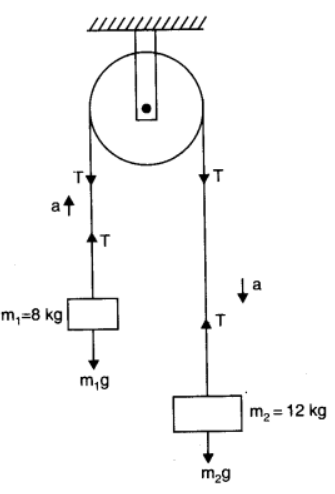Smaller mass, m1 = 8 kg
Larger mass, m2 = 12 kg
Tension in the string = T
Mass m2, owing to its weight, moves downward with acceleration a,and mass m1 moves upward.
Applying Newton’s second law of motion to the system of each mass:

For mass m1:
The equation of motion can be written as:
T – m1g = ma … (i)

For mass m2:
The equation of motion can be written as:
m2g – T = m2a … (ii)

Adding equations (i) and (ii), we get:
(m2 - m1)g = (m1 + m2)a
∴ a = ( (m2 - m1) / (m1 + m2) )g    ....(iii)
= (12 - 8) / (12 + 8) × 10  =  4 × 10 / 20  =  2 ms-2
Therefore, the acceleration of the masses is 2 m/s2.
Substituting the value of a in equation (ii), we get:
m2g - T = m2(m2 - m1)g / (m1 + m2)
T = (m2 - (m22 - m1m2) / (m1 + m2) )g
= 2m1m2g / (m1 + m2)
= 2 × 12 × 8 × 10 / (12 + 8)
= 96 N
Therefore, the tension in the string is 96 N.

Question 17. A nucleus is at rest in the laboratory frame of reference. Show that if it disintegrates into two smaller nuclei the products must move in opposite directions.

Solution :
Let m, m1, and m2 be the respective masses of the parent nucleus and the two daughter nuclei. The parent nucleus is at rest.
Initial momentum of the system (parent nucleus) = 0
Let v1 and v2 be the respective velocities of the daughter nuclei having masses m1 and m2.
Total linear momentum of the system after disintegration = m1v1 + m2v2
According to the law of conservation of momentum:
Total initial momentum = Total final momentum
0 = m1v1 + m2v2
v1 = -m2v2 / m1
Here, the negative sign indicates that the fragments of the parent nucleus move in directions opposite to each other.

Question 18. Two billiard balls each of mass 0.05 kg moving in opposite directions with speed 6 m s–1 collide and rebound with the same speed. What is the impulse imparted to each ball due to the other?

Solution :
Mass of each ball = 0.05 kg
Initial velocity of each ball = 6 m/s
Magnitude of the initial momentum of each ball, pi = 0.3 kg m/s
After collision, the balls change their directions of motion without changing the magnitudes of their velocity.
Final momentum of each ball, pf = –0.3 kg m/s
Impulse imparted to each ball = Change in the momentum of the system
= pf – pi
= –0.3 – 0.3 = –0.6 kg m/s
The negative sign indicates that the impulses imparted to the balls are opposite in direction.

Question 19. A shell of mass 0.020 kg is fired by a gun of mass 100 kg. If the muzzle speed of the shell is 80 m s–1, what is the recoil speed of the gun?

Solution :
Mass of the gun, M = 100 kg
Mass of the shell, m = 0.020 kg
Muzzle speed of the shell, v = 80 m/s
Recoil speed of the gun = V
Both the gun and the shell are at rest initially.
Initial momentum of the system = 0
Final momentum of the system = mv – MV
Here, the negative sign appears because the directions of the shell and the gun are opposite to each other.
According to the law of conservation of momentum:
Final momentum = Initial momentum
mv – MV = 0
∴ V = mv / M
= 0.020 × 80 / (100 × 1000) = 0.016 m/s

Question 20. A batsman deflects a ball by an angle of 45° without changing its initial speed which is equal to 54 km/h. What is the impulse imparted to the ball? (Mass of the ball is 0.15 kg.)

Solution :

The given situation can be represented as shown in the following figure.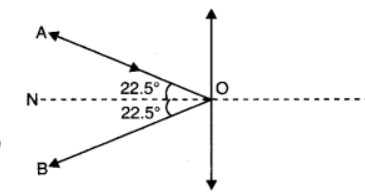Where,
AO = Incident path of the ball
OB = Path followed by the ball after deflection
∠AOB = Angle between the incident and deflected paths of the ball = 45°
∠AOP = ∠BOP = 22.5° = θ
Initial and final velocities of the ball = v
Horizontal component of the initial velocity = vcos θ along RO
Vertical component of the initial velocity = vsin θ along PO
Horizontal component of the final velocity = vcos θ along OS
Vertical component of the final velocity = vsin θ along OP
The horizontal components of velocities suffer no change. The vertical components of velocities are in the opposite directions.
∴ Impulse imparted to the ball = Change in the linear momentum of the ball
= mvCosθ - (-mvCosθ)  =  2mvCosθ
Mass of the ball, m = 0.15 kg
Velocity of the ball, v = 54 km/h = 15 m/s
∴ Impulse = 2 × 0.15 × 15 cos 22.5° = 4.16 kg m/s

Question 21. A stone of mass 0.25 kg tied to the end of a string is whirled round in a circle of radius 1.5 m with a speed of 40 rev./min in a horizontal plane. What is the tension in the string? What is the maximum speed with which the stone can be whirled around if the string can withstand a maximum tension of 200 N?

Solution :
Mass of the stone, m = 0.25 kg
Radius of the circle, r = 1.5 m
Number of revolution per second, n = 40 / 60 = 2 / 3 rps
Angular velocity, ω = v / r = 2πn
The centripetal force for the stone is provided by the tension T, in the string, i.e.,
T = FCentripetal
= mv2 / r = mrω = mr(2πn)2
= 0.25 × 1.5 × (2 × 3.14 × (2/3) )2
= 6.57 N
Maximum tension in the string, Tmax = 200 N
Tmax = mv2max / r
∴ vmax = (Tmax × r  / m)1/2
= (200 × 1.5 / 0.25)1/2
= (1200)1/2 = 34.64 m/s
Therefore, the maximum speed of the stone is 34.64 m/s.

Question 22. If, in Exercise 5.21, the speed of the stone is increased beyond the maximum permissible value, and the string breaks suddenly, which of the following correctly describes the trajectory of the stone after the string breaks:
a. the stone moves radially outwards,
b. the stone flies off tangentially from the instant the string breaks,
c. the stone flies off at an angle with the tangent whose magnitude depends on the speed of the particle ?

Solution :
b.  the stone flies off tangentially from the instant the string breaks,
When the string breaks, the stone will move in the direction of the velocity at that instant. According to the first law of motion, the direction of velocity vector is tangential to the path of the stone at that instant. Hence, the stone will fly off tangentially from the instant the string breaks.

Question23. Explain why
a. a horse cannot pull a cart and run in empty space,
b. passengers are thrown forward from their seats when a speeding bus stops suddenly,
c. it is easier to pull a lawn mower than to push it,
d. a cricketer moves his hands backwards while holding a catch.

Solution :
a. While trying to pull a cart, ahorse pushes the ground backward with some force. The ground in turn exerts an equal and opposite reaction force upon the feet of the horse. This reaction force causes the horse to move forward.
An empty space is devoid of any such reaction force. Therefore, a horse cannot pull a cart and run in empty space.

b. This is due to inertia of motion. When a speeding bus stops suddenly, the lower part of a passenger's body, which is in contact with the seat, suddenly comes to rest. However, the upper part tends to remain in motion (as per the first law of motion). As a result, the passenger's upper body is thrown forward in the direction in which the bus was moving.

c. When the lawnmower is pulled, the vertical component of the applied force acts upwards. This reduces the effective weight of the lawnmower. When the lawn mower is pushed, the vertical component acts in the direction of the weight of the mower. Therefore, there is an increase in the weight of the mower. So, it is easier to pull a lawnmower than to push it.
d. According to Newton’s second law of motion, we have the equation of motion:
F = ma = m ∆v /∆t      ...(i)
Where,
F = Stopping force experienced by the cricketer as he catches the ball
m = Mass of the ball
Δt = Time of impact of the ball with the hand
It can be inferred from equation (i) that the impact force is inversely proportional to the impact time, i.e.,
F  ∝1/ Δt      ....(ii)
Equation (ii) shows that the force experienced by the cricketer decreases if the time of impact increases and vice versa.
While taking a catch, a cricketer moves his hand backward so as to increase the time of impact (Δt). This is turn results in the decrease in the stopping force, thereby preventing the hands of the cricketer from getting hurt.

Question 24. Figure 5.17 shows the position-time graph of a body of mass 0.04 kg. Suggest a suitable physical context for this motion. What is the time between two consecutive impulses received by the body? What is the magnitude of each impulse?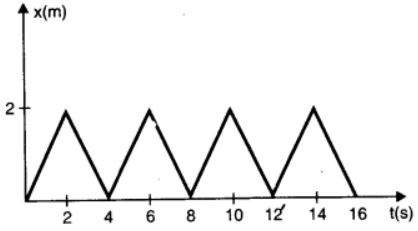Solution :
A ball rebounding between two walls located between at x = 0 and x = 2 cm; after every 2 s, the ball receives an impulse of magnitude 0.08 × 10–2 kg m/s from the walls
The given graph shows that a body changes its direction of motion after every 2 s. Physically, this situation can be visualized as a ball rebounding to and fro between two stationary walls situated between positions x = 0 and x = 2 cm. Since the slope of the x-t graph reverses after every 2 s, the ball collides with a wall after every 2 s. Therefore, ball receives an impulse after every 2 s.
Mass of the ball, m = 0.04 kg
The slope of the graph gives the velocity of the ball. Using the graph, we can calculate initial velocity (u) as:u = (2 - 0) × 10-2 / (2 - 0)  =  10-2 m/s
Velocity of the ball before collision, u = 10–2 m/s
Velocity of the ball after collision, v = –10–2 m/s
(Here, the negative sign arises as the ball reverses its direction of motion.)
Magnitude of impulse = Change in momentum
= | mv - mu |
= | 0.04 (v - u) |
= | 0.04 (-10-2 - 10-2) |
= 0.08 × 10-2 kg m/s

Question 25.Figure 5.18 shows a man standing stationary with respect to a horizontal conveyor belt that is accelerating with 1 m s–2. What is the net force on the man? If the coefficient of static friction between the man’s shoes and the belt is 0.2, up to what acceleration of the belt can the man continue to be stationary relative to the belt? (Mass of the man = 65 kg.)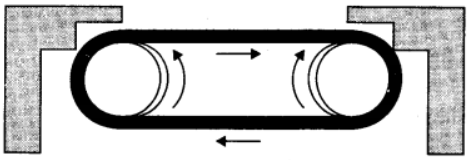Solution :
Mass of the man, m = 65 kg
Acceleration of the belt, a = 1 m/s2
Coefficient of static friction, μ = 0.2
The net force F, acting on the man is given by Newton’s second law of motion as:
Fnet = ma = 65 × 1 = 65 N
The man will continue to be stationary with respect to the conveyor belt until the net force on the man is less than or equal to the frictional force fs, exerted by the belt, i.e.,
F'net = fs
ma' = μmg
∴ a' = 0.2 × 10 = 2 m/s2
Therefore, the maximum acceleration of the belt up to which the man can stand stationary is 2 m/s2.

Question 26. A stone of mass m tied to the end of a string revolves in a vertical circle of radius R. The net forces at the lowest and highest points of the circle directed vertically downwards are: [Choose the correct alternative]

 Lowest Point Highest Point (a) mg – T1 mg + T2 (b) mg + T1 mg – T2 (c) mg + T1 – (mv12) / R mg – T2 + (mv12) / R (d) mg – T1 – (mv12) / R mg + T2 + (mv12) / R

T1 and V1 denote the tension and speed at the lowest point. T2 and v2 denote corresponding values at the highest point.

Solution :
(a) The free body diagram of the stone at the lowest point is shown in the following figure.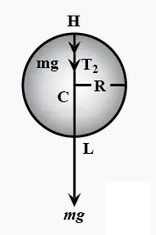According to Newton’s second law of motion, the net force acting on the stone at this point is equal to the centripetal force, i.e.,
Fnet = T - mg = mv12 / R   ....(i)
Where, v1 = Velocity at the lowest point

The free body diagram of the stone at the highest point is shown in the following figure.Using Newton’s second law of motion, we have:
T + mg = mv22 / R    ...(ii)
Where, v2 = Velocity at the highest point
It is clear from equations (i) and (ii) that the net force acting at the lowest and the highest points are respectively (T – mg) and (T + mg).

Question27. A helicopter of mass 1000 kg rises with a vertical acceleration of 15 m s–2. The crew and the passengers weigh 300 kg. Give the magnitude and direction of the
a. force on the floor by the crew and passengers,
b. action of the rotor of the helicopter on the surrounding air,
c. force on the helicopter due to the surrounding air.

Solution :
a. Mass of the helicopter, mh = 1000 kg
Mass of the crew and passengers, mp = 300 kg
Total mass of the system, m = 1300 kg
Acceleration of the helicopter, a = 15 m/s2
Using Newton’s second law of motion, the reaction force R, on the system by the floor can be calculated as:
R – mpg = ma
= mp(g + a)
= 300 (10 + 15) = 300 × 25
= 7500 N
Since the helicopter is accelerating vertically upward, the reaction force will also be directed upward. Therefore, as per Newton’s third law of motion, the force on the floor by the crew and passengers is 7500 N, directed downward.

b. Using Newton’s second law of motion, the reaction force R’, experienced by the helicopter can be calculated as:
R' - mg = ma
= m(g + a)
= 1300 (10 + 15) = 1300 × 25
= 32500 N
The reaction force experienced by the helicopter from the surrounding air is acting upward. Hence, as per Newton’s third law of motion, the action of the rotor on the surrounding air will be 32500 N, directed downward.

c.  The force on the helicopter due to the surrounding air is 32500 N, directed upward.

Question 28. A stream of water flowing horizontally with a speed of 15 m s–1 gushes out of a tube of cross-sectional area 10–2 m2, and hits a vertical wall nearby. What is the force exerted on the wall by the impact of water, assuming it does not rebound?

Solution :
Speed of the water stream, v = 15 m/s
Cross-sectional area of the tube, A = 10–2 m2
Volume of water coming out from the pipe per second,
V = Av = 15 × 10–2 m3/s
Density of water, ρ = 103 kg/m3
Mass of water flowing out through the pipe per second = ρ × V = 150 kg/s
The water strikes the wall and does not rebound. Therefore, the force exerted by the water on the wall is given by Newton’s second law of motion as:
F = Rate of change of momentum = ∆P / ∆t
= mv / t
= 150 × 15 = 2250 N

Question 29. Ten one-rupee coins are put on top of each other on a table. Each coin has a mass m. Give the magnitude and direction of
a. the force on the 7th coin (counted from the bottom) due to all the coins on its top,
b. the force on the 7th coin by the eighth coin,
c. the reaction of the 6th coin on the 7th coin.

Solution :
a. Force on the seventh coin is exerted by the weight of the three coins on its top.
Weight of one coin = mg
Weight of three coins = 3mg
Hence, the force exerted on the 7th coin by the three coins on its top is 3mg. This force acts vertically downward.

b. Force on the seventh coin by the eighth coin is because of the weight of the eighth coin and the other two coins (ninth and tenth) on its top.
Weight of the eighth coin = mg
Weight of the ninth coin = mg
Weight of the tenth coin = mg
Total weight of these three coins = 3mg
Hence, the force exerted on the 7th coin by the eighth coin is 3mg. This force acts vertically downward.

c. The 6th coin experiences a downward force because of the weight of the four coins (7th, 8th, 9th, and 10th) on its top.
Therefore, the total downward force experienced by the 6th coin is 4mg.
As per Newton’s third law of motion, the 6th coin will produce an equal reaction force on the 7th coin, but in the opposite direction. Hence, the reaction force of the 6th coin on the 7th coin is of magnitude 4mg. This force acts in the upward direction.

Question 30. An aircraft executes a horizontal loop at a speed of 720 km/h with its wings banked at 15°. What is the radius of the loop?

Solution :
Speed of the aircraft, v = 720 km/h = 720 × 5 / 18  =  200 m/s
Acceleration due to gravity, g = 10 m/s2
Angle of banking, θ = 15°
For radius r, of the loop, we have the relation:
tan θ = v2 / rg
r = v2 / g tan θ
= 2002 / (10 × tan 15)
= 4000 / 0.26
= 14925.37 m
= 14.92 km

Question 31. A train runs along an unbanked circular track of radius 30 m at a speed of 54 km/h. The mass of the train is 106 kg. What provides the centripetal force required for this purpose – The engine or the rails? What is the angle of banking required to prevent wearing out of the rail?

Solution :
Radius of the circular track, r = 30 m
Speed of the train, v = 54 km/h = 15 m/s
Mass of the train, m = 106 kg
The centripetal force is provided by the lateral thrust of the rail on the wheel. As per Newton’s third law of motion, the wheel exerts an equal and opposite force on the rail. This reaction force is responsible for the wear and rear of the rail
The angle of banking θ, is related to the radius (r) and speed (v) by the relation:
tan θ = v2 / rg
= 152 / (30 × 10)
= 225 / 300
θ = tan-1 (0.75) = 36.870
Therefore, the angle of banking is about 36.87°.

Question 32. A block of mass 25 kg is raised by a 50 kg man in two different ways as shown in Fig. 5.19. What is the action on the floor by the man in the two cases? If the floor yields to a normal force of 700 N, which mode should the man adopt to lift the block without the floor yielding?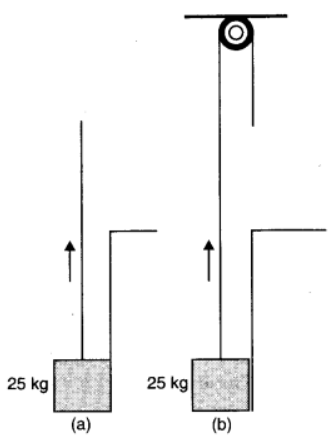Solution :

750N and 250 N in the respective cases; Method (b)
Mass of the block, m = 25 kg
Mass of the man, M = 50 kg
Acceleration due to gravity, g = 10 m/s2
Force applied on the block, F = 25 × 10 = 250 N
Weight of the man, W = 50 × 10 = 500 N

Case (a): When the man lifts the block directly
In this case, the man applies a force in the upward direction. This increases his apparent weight.
∴Action on the floor by the man = 250 + 500 = 750 N

Case (b): When the man lifts the block using a pulley
In this case, the man applies a force in the downward direction. This decreases his apparent weight.
∴Action on the floor by the man = 500 – 250 = 250 N

If the floor can yield to a normal force of 700 N, then the man should adopt the second method to easily lift the block by applying lesser force.

Question 33. A monkey of mass 40 kg climbs on a rope (Fig. 5.20) which can stand a maximum tension of 600 N. In which of the following cases will the rope break: the monkey
a. climbs up with an acceleration of 6 m s–2
b. climbs down with an acceleration of 4 m s–2
c. climbs up with a uniform speed of 5 m s–1
d. falls down the rope nearly freely under gravity?
(Ignore the mass of the rope).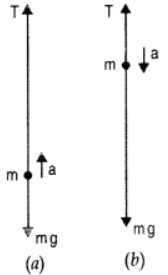Solution :
Case (a)
Mass of the monkey, m = 40 kg
Acceleration due to gravity, g = 10 m/s
Maximum tension that the rope can bear, Tmax = 600 N
Acceleration of the monkey, a = 6 m/s2 upward
Using Newton’s second law of motion, we can write the equation of motion as:
T – mg = ma
∴T = m(g + a)
= 40 (10 + 6)
= 640 N
Since T > Tmax, the rope will break in this case.

Case (b)
Acceleration of the monkey, a = 4 m/s2 downward
Using Newton’s second law of motion, we can write the equation of motion as:
mg – T = ma
∴T = m (g – a)
= 40(10 – 4)
= 240 N
Since T < Tmax, the rope will not break in this case.

Case (c)
The monkey is climbing with a uniform speed of 5 m/s. Therefore, its acceleration is zero, i.e., a = 0.
Using Newton’s second law of motion, we can write the equation of motion as:
T – mg = ma
T – mg = 0
∴T = mg
= 40 × 10
= 400 N
Since T < Tmax, the rope will not break in this case.

Case (d)
When the monkey falls freely under gravity, its will acceleration become equal to the acceleration due to gravity, i.e., a = g
Using Newton’s second law of motion, we can write the equation of motion as:
mg – T = mg
∴T = m(g – g) = 0
Since T < Tmax, the rope will not break in this case.

Question 34.  Two bodies A and B of masses 5 kg and 10 kg in contact with each other rest on a table against a rigid wall (Fig. 5.21). The coefficient of friction between the bodies and the table is 0.15. A force of 200 N is applied horizontally to A. What are

a. the reaction of the partition

b. the action-reaction forces between A and B? What happens when the wall is removed? Does the answer to (b) change, when the bodies are in motion? Ignore the difference between μs and μk.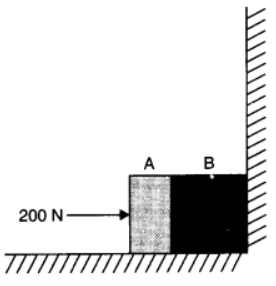Solution :
a.  Mass of body A, mA = 5 kg Mass of body B, mB = 10 kg
Applied force, F = 200 N
Coefficient of friction, μs = 0.15
The force of friction is given by the relation:
fs = μ (mA + mB)g
= 0.15 (5 + 10) × 10
= 1.5 × 15 = 22.5 N leftward
Net force acting on the partition = 200 – 22.5 = 177.5 N rightward
As per Newton’s third law of motion, the reaction force of the partition will be in the direction opposite to the net applied force.
Hence, the reaction of the partition will be 177.5 N, in the leftward direction.

b. Force of friction on mass A:
fA = μmAg
= 0.15 × 5 × 10 = 7.5 N leftward
Net force exerted by mass A on mass B = 200 – 7.5 = 192.5 N rightward
As per Newton’s third law of motion, an equal amount of reaction force will be exerted by mass B on mass A, i.e., 192.5 N acting leftward.
When the wall is removed, the two bodies will move in the direction of the applied force.
Net force acting on the moving system = 177.5 N
The equation of motion for the system of acceleration a,can be written as:
Net force = (mA + mB) a
∴ a = Net force / (mA + mB)
= 177.5 / (5 + 10) = 177.5 / 15 = 11.83 ms-2
Net force causing mass A to move:
FA = mAa
= 5 × 11.83 = 59.15 N
Net force exerted by mass A on mass B = 192.5 – 59.15 = 133.35 N
This force will act in the direction of motion. As per Newton’s third law of motion, an equal amount of force will be exerted by mass B on mass A, i.e., 133.3 N, acting opposite to the direction of motion.

Question 35. A block of mass 15 kg is placed on a long trolley. The coefficient of static friction between the block and the trolley is 0.18. The trolley accelerates from rest with 0.5 m s–2 for 20 s and then moves with uniform velocity. Discuss the motion of the block as viewed by (a) a stationary observer on the ground, (b) an observer moving with the trolley.

Solution :
a.  Mass of the block, m = 15 kg
Coefficient of static friction, μ = 0.18
Acceleration of the trolley, a = 0.5 m/s2
As per Newton’s second law of motion, the force (F) on the block caused by the motion of the trolley is given by the relation:
F = ma = 15 × 0.5 = 7.5 N
This force is acted in the direction of motion of the trolley.
Force of static friction between the block and the trolley:
f = μmg
= 0.18 × 15 × 10 = 27 N
The force of static friction between the block and the trolley is greater than the applied external force. Hence, for an observer on the ground, the block will appear to be at rest.
When the trolley moves with uniform velocity there will be no applied external force. Only the force of friction will act on the block in this situation.

b.  An observer, moving with the trolley, has some acceleration. This is the case of non-inertial frame of reference. The frictional force, acting on the trolley backward, is opposed by a pseudo force of the same magnitude. However, this force acts in the opposite direction. Thus, the trolley will appear to be at rest for the observer moving with the trolley.

Question 36. The rear side of a truck is open and a box of 40 kg mass is placed 5 m away from the open end as shown in Fig. 5.22. The coefficient of friction between the box and the surface below it is 0.15. On a straight road, the truck starts from rest and accelerates with 2 m s–2. At what distance from the starting point does the box fall off the truck? (Ignore the size of the box).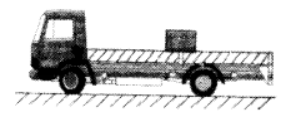Solution :
Mass of the box, m = 40 kg
Coefficient of friction, μ = 0.15
Initial velocity, u = 0
Acceleration, a = 2 m/s2
Distance of the box from the end of the truck, s' = 5 m
As per Newton’s second law of motion, the force on the box caused by the accelerated motion of the truck is given by:
F = ma
= 40 × 2 = 80 N
As per Newton’s third law of motion, a reaction force of 80 N is acting on the box in the backward direction. The backward motion of the box is opposed by the force of friction f, acting between the box and the floor of the truck. This force is given by:
f = μmg
= 0.15 × 40 × 10 = 60 N
∴Net force acting on the block:
Fnet = 80 – 60 = 20 N backward
The backward acceleration produced in the box is given by:
aback = Fnet / m  =  20 / 40 = 0.5 ms-2
Using the second equation of motion, time t can be calculated as:
s' = ut + (1/2)abackt2
5 = 0 + (1/2) × 0.5 × t2
∴ t = √20 s
Hence, the box will fall from the truck after √
20 s from start.
The distance s, travelled by the truck in √
20 s is given by the relation:
s = ut + (1/2)at2
= 0 + (1/2) × 2 × (√20)2
= 20 m

Question 37. A disc revolves with a speed of 100 / 3 rev / min, and has a radius of 15 cm. Two coins are placed at 4 cm and 14 cm away from the centre of the record. If the co-efficient of friction between the coins and the record is 0.15, which of the coins will revolve with the record?

Solution :
Coin placed at 4 cm from the centre
Mass of each coin = m
Radius of the disc, r = 15 cm = 0.15 m
Frequency of revolution, ν = 100 / 3 rev/min = 100 / (3 × 60) = 5 / 9 rev/s
Coefficient of friction, μ = 0.15
In the given situation, the coin having a force of friction greater than or equal to the centripetal force provided by the rotation of the disc will revolve with the disc. If this is not the case, then the coin will slip from the disc.

Coin placed at 4 cm:
Radius of revolution, r' = 4 cm = 0.04 m
Angular frequency, ω = 2πν = 2 × (22/7) × (5/9) = 3.49 s-1
Frictional force, f = μmg = 0.15 × m × 10 = 1.5m N
Centripetal force on the coin:
Fcent. = mr'ω2
= m × 0.04 × (3.49)2
= 0.49m N
Since f > Fcent, the coin will revolve along with the record.

Coin placed at 14 cm:
Radius, r" = 14 cm = 0.14 m
Angular frequency, ω = 3.49 s–1
Frictional force, f' = 1.5m N
Centripetal force is given as:
Fcent. = mr"ω2
= m × 0.14 × (3.49)2
= 1.7m N
Since f < Fcent., the coin will slip from the surface of the record.

Question 38. You may have seen in a circus a motorcyclist driving in vertical loops inside a ‘death-well’ (a hollow spherical chamber with holes, so the spectators can watch from outside). Explain clearly why the motorcyclist does not drop down when he is at the uppermost point, with no support from below. What is the minimum speed required at the uppermost position to perform a vertical loop if the radius of the chamber is 25 m?

Solution :
In a death-well, a motorcyclist does not fall at the top point of a vertical loop because both the force of normal reaction and the weight of the motorcyclist act downward and are balanced by the centripetal force. This situation is shown in the following figure.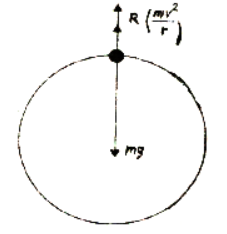The net force acting on the motorcyclist is the sum of the normal force (FN) and the force due to gravity (Fg = mg).
The equation of motion for the centripetal acceleration ac, can be written as:
Fnet = mac
FN + Fg = mac
FN + mg = mv2 / r
Normal reaction is provided by the speed of the motorcyclist. At the minimum speed (vmin), FN = 0
mg = mvmin2 / r
∴ vmin = √rg

= √25 X √10 = 15.8 m/s

Question 39. A 70 kg man stands in contact against the inner wall of a hollow cylindrical drum of radius 3 m rotating about its vertical axis with 200 rev/min. The coefficient of friction between the wall and his clothing is 0.15. What is the minimum rotational speed of the cylinder to enable the man to remain stuck to the wall (without falling) when the floor is suddenly removed?

Solution :
Mass of the man, m = 70 kg
Radius of the drum, r = 3 m
Coefficient of friction, μ = 0.15
Frequency of rotation, ν = 200 rev/min = 200 / 60 = 10 / 3 rev/s
The necessary centripetal force required for the rotation of the man is provided by the normal force (FN).
When the floor revolves, the man sticks to the wall of the drum. Hence, the weight of the man (mg) acting downward is balanced by the frictional force
(f = μFN) acting upward.
Hence, the man will not fall until:
mg < f
mg < μFN = μmrω2
g < μrω2
ω > (g / μr)1/2
The minimum angular speed is given as:
ωmin = (g / μr)1/2
= ( 10 / (0.15 X 3) )1/2  =  4.71 rad s-1

Question 40. A thin circular loop of radius R rotates about its vertical diameter with an angular frequency ω. Show that a small bead on the wire loop remains at its lowermost point for ω ≤ √g/R. What is the angle made by the radius vector joining the centre to the bead with the vertical downward direction for ω = √2g/R? Neglect friction.

Solution :
Let the radius vector joining the bead with the centre make an angle θ, with the vertical downward direction.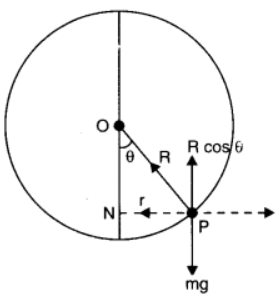OP = R = Radius of the circle
N = Normal reaction
The respective vertical and horizontal equations of forces can be written as:
mg = N Cosθ ... (i)
mlω2 = N Sinθ … (ii)

In ΔOPQ, we have:
Sin θ = l / R
l = R Sinθ … (iii)
Substituting equation (iii) in equation (ii), we get:
m(R Sinθ) ω2 = N Sinθ
mR ω2 = N ... (iv)
Substituting equation (iv) in equation (i), we get:
mg = mR ω2 Cosθ
Cosθ = g / Rω2    ...(v)
Since cosθ ≤ 1, the bead will remain at its lowermost point for g / Rω2 ≤ 1, i.e., for ω ≤ (g / R)1/2
For ω = (2g / R)1/2  or ω2 = 2g / R   .....(vi)

On equating equations (v) and (vi), we get:
2g / R = g / RCos θ
Cos θ = 1 / 2
∴ θ = Cos-1(0.5)  =  600